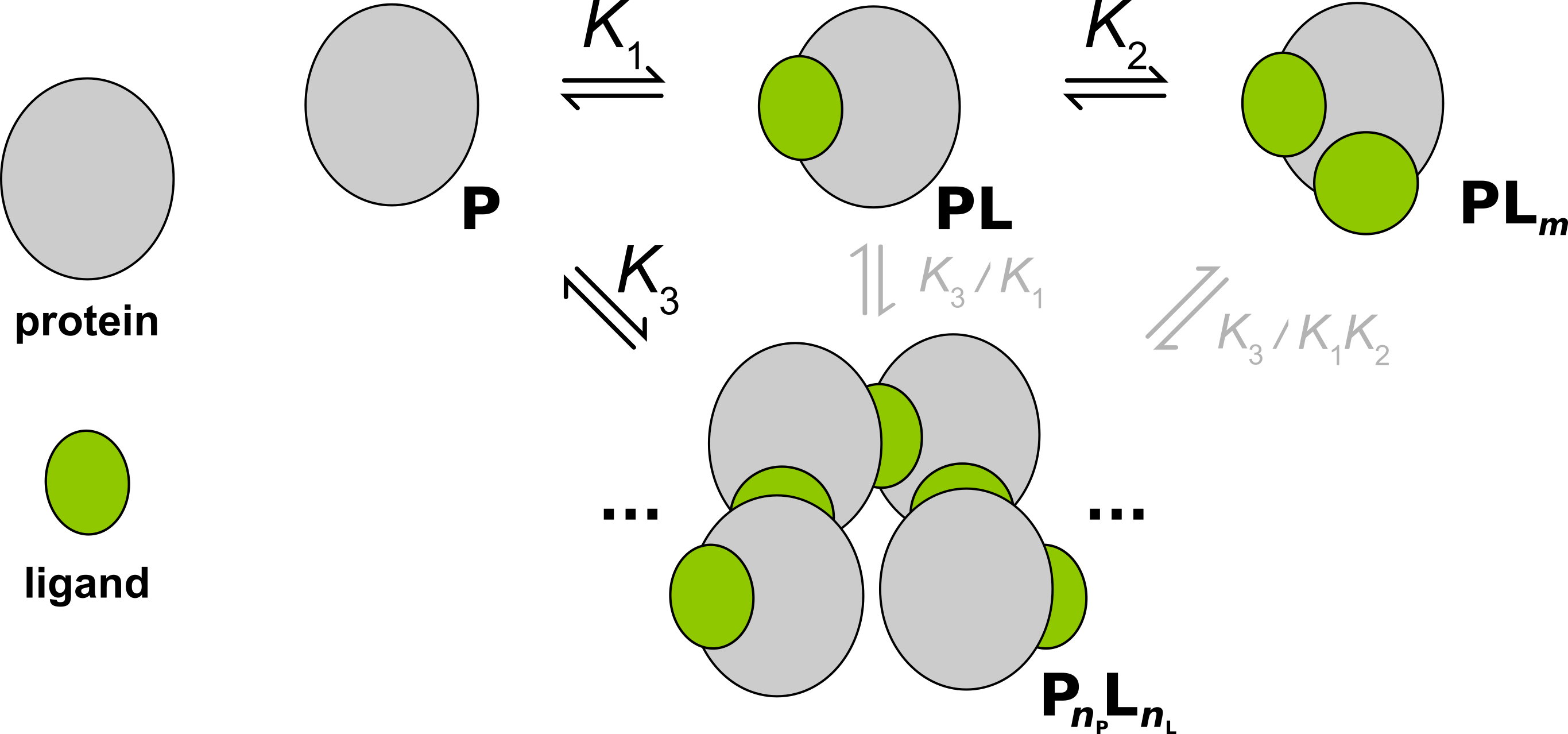# Assembly Auto Inhibition¶

• Ligand binding that promotes protein oligomerization, which is auto-inhibited by saturation of ligand (related to the prozone effect).
• Model contributed by: Martin Rennie, PhD
• Rennie & Crowley (2019). ChemPhysChem (link).
• indiv_models.AssemblyAutoInhibition

## Scheme¶Illustrated with $$m=2$$, $$n_L=5$$, $$n_P=4$$.

## Parameters¶

parameter variable parameter name class
macroscopic association constant for binding of the first ligand to the protein monomer (M-1) $$K_{1}$$ Klig1 thermodynamic
average* association constant for binding of the remaining ligands to the protein monomer (M-1) $$K_{2}$$ Klig2 thermodynamic
average* association constant for formation of the protein oligomer (M-1) $$K_{3}$$ Kolig thermodynamic
enthalpy change for binding of the first ligand to the protein monomer $$\Delta H_{1}$$ dHlig1 thermodynamic
enthalpy change for binding of the remaining ligands to the protein monomer $$\Delta H_{2}$$ dHlig2 thermodynamic
enthalpy change for formation of the protein oligomer $$\Delta H_{3}$$ dHolig thermodynamic
stoichiometry of ligands in the saturated protein monomer, must be ≥2 $$m$$ m thermodynamic
stoichiometry of ligands in the protein oligomer $$n_{L}$$ n_lig thermodynamic
stoichiometry of proteins in the protein oligomer $$n_{P}$$ n_prot thermodynamic
fraction competent protein fx_prot_competent nuisance
fraction competent ligand fx_lig_competent nuisance
slope of heat of dilution dilution_heat nuisance
intercept of heat of dilution dilution_intercept nuisance

*equilibrium constants for the higher order equilibria are “averaged” using $$\sqrt[N]{K}$$, where $$N$$ is the order of the equilibrium, such that the units are M-1 (see Rennie & Crowley (2019). ChemPhysChem (link))

## Species¶

$[P_{T}]_{i} = [P]_{i} + [PL]_{i} + [PL_{m}]_{i} + n_{P}[P_{olig}]_{i}$
$[L_{T}]_{i} = [L]_{i} + [PL]_{i} + m[PL_{m}]_{i} + n_{L}[P_{olig}]_{i}$
$[PL]_{i} = K_{1}[P]_{i}[L]_{i}$
$[PL_{2}]_{i} = K_{1}K_{2}^{m-1}[P]_{i}[L]_{i}^{m}$
$[P_{olig}]_{i} = K_{3}^{n_{L}+n_{P}-1}[P]_{i}^{n_{P}}[L]_{i}^{n_{L}}$

## Heat¶

$\begin{split}q_{i} = V_{cell}\Big ( \Delta H_{1}^{\circ}([PL]_{i} - [PL]_{i-1}(1-v_{i}/V_{cell})) \\ + (\Delta H_{1}^{\circ} + \Delta H_{2}^{\circ})([PL_{2}]_{i} - [PL_{2}]_{i-1}(1 - v_{i}/V_{cell})) \\ + \Delta H_{3}^{\circ}([P_{olig}]_{i} - [P_{olig}]_{i-1}(1 - v_{i}/V_{cell})) \Big ) + q_{dil}\end{split}$

where: $$[P_{T}]_{i}$$ is the total cell concentration of protein at the $$i^\text{th}$$ injection (independent variable); $$[L_{T}]_{i}$$ is the total cell concentration of ligand at the $$i^\text{th}$$ injection (independent variable); $$V_{cell}$$ is the volume of the cell; $$v_{i}$$ is the volume of the $$i^\text{th}$$ injection; $$q_{i}$$ is the heat generated from the $$i^\text{th}$$ injection; $$q_{dil}$$ is the heat of dilution.